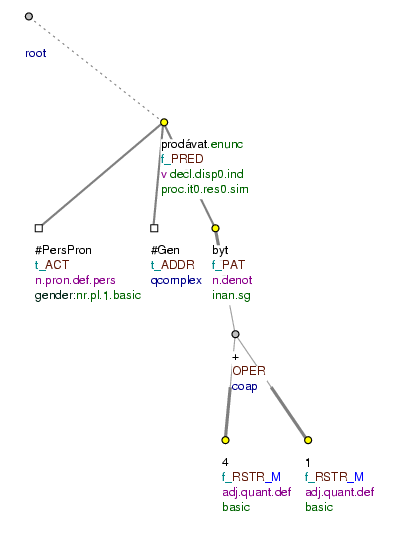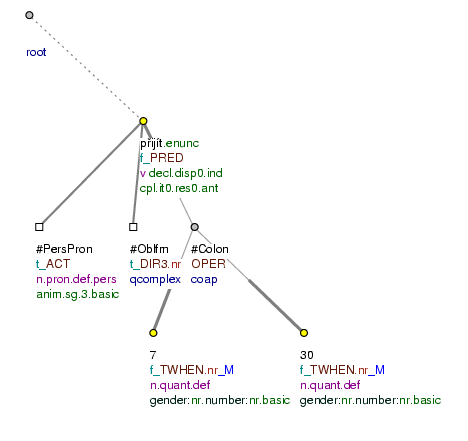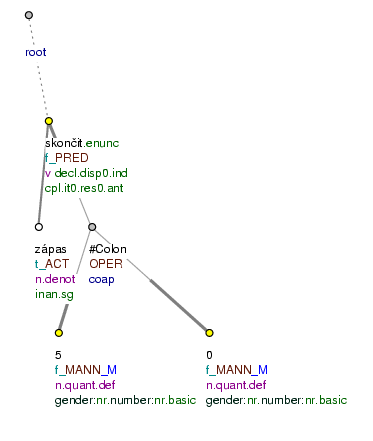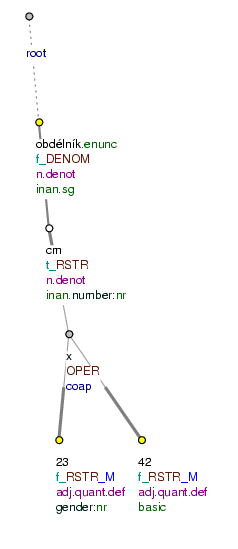### 11.1. Mathematical operations

Mathematical operations are constructions used for expressing proportion (division), multiplication, addition and subtraction.

Constructions with the meaning of mathematical operations are analyzed as paratactic structures (see Section 6, "Parataxis"). The relation between the two parts of a paratactic structure is expressed by the functor `OPER` (see Section 12.3, "Functor for mathematical operations and intervals (`OPER`)") assigned to the root of the paratactic structure. The root of such a paratactic structure is the node representing the given operator (for more on operators, see Section 16.2, "Operators").

The nodes representing operands of a given mathematical operation are the terminal members of the paratactic structure. The value of their `is_member` attribute is `1`. Nodes that are neither operands of a mathematical operation nor delimit an interval are assigned the `0` value in their `is_member` attribute (see also Table 6.3, "Values of the `is_member` attribute").

The functor of the operands corresponds to the position of the whole structure in the sentence, and is usually the same for both (all) of them.

Examples:

Prodáváme byt 4+1. (=We are selling an appartment 4+1) Fig. 8.199

Přišel v 7:30. (=He came at 7:30) Fig. 8.200

Zápas skončil 5:0. (=The match ended 5:0) Fig. 8.201

obdélník 23x42 cm (=a square 23x42 cm) Fig. 8.202

10 mínus 2 je 8. (10 minus 2 is 8)

!!! Individual types of mathematical operations will be distinguished by various subfunctors of the `OPER` functor in the future.

!!! Time in the form 7 : 30 (with a colon) is represented as a mathematical operation (paratactic structure), time in the form 7.30 (with a period) is represented by a single node with the t-lemma 7.30.

Figure 8.199. Mathematical operationProdáváme byt 4+1. (=lit. (We) are_selling flat 4+1)

Figure 8.200. Mathematical operationPřišel v 7:30. (=lit. (He) came at 7:30)

Figure 8.201. Mathematical operationZápas skončil 5 : 0. (=lit. Match ended 5:0)

Figure 8.202. Mathematical operationobdélník 23 x 42 cm (=lit. square 23 x 42 cm)